#### Printable version

Detailed instructions for working a group puzzle of type 'tpsmith'

The 12 elements in each group of this type of puzzle are: 0, 1, 2, 3, 4, 5, 6, 7, 8, 9, A, and B.

Every group in the puzzle must contain exactly this set of elements.

####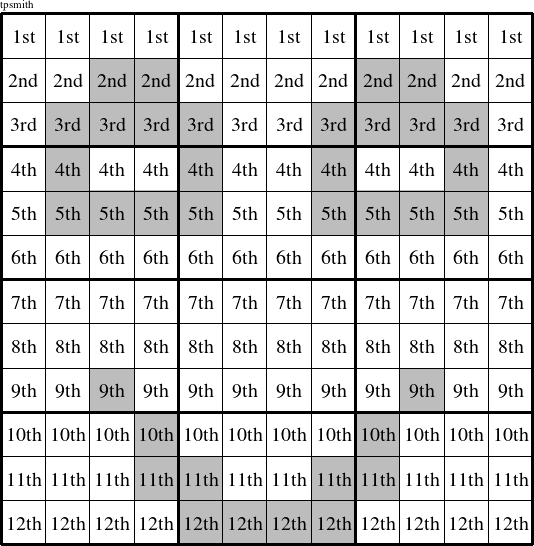In a tpsmith puzzle, each row is a group numbered as shown in the figure above.

####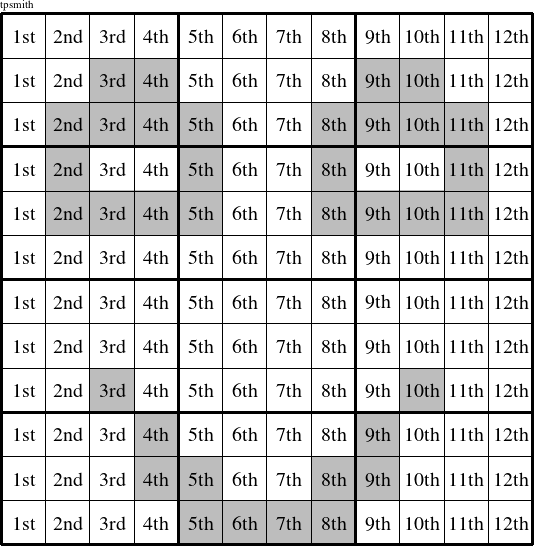In a tpsmith puzzle, each column is a group numbered as shown in the figure above.

####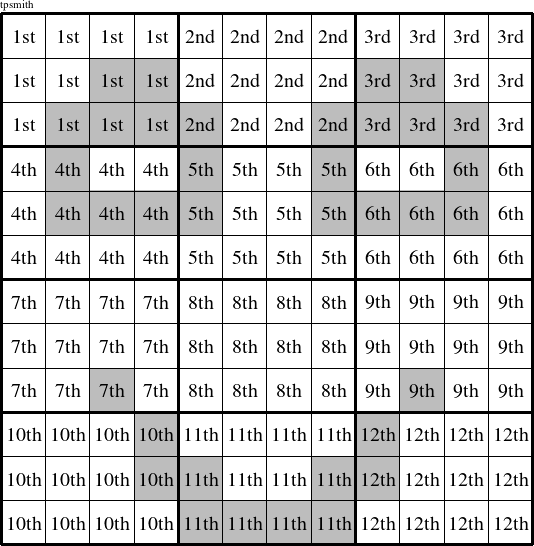In a tpsmith puzzle, each 4x3 rectangle is a group numbered as shown in the figure above.

####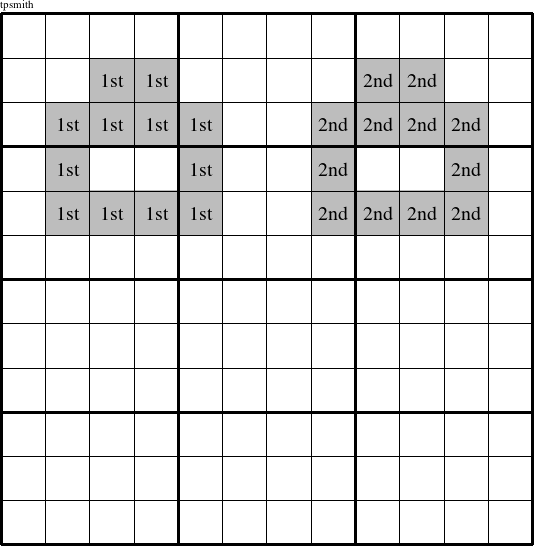In a tpsmith puzzle, each eye is a group numbered as shown in the figure above.

####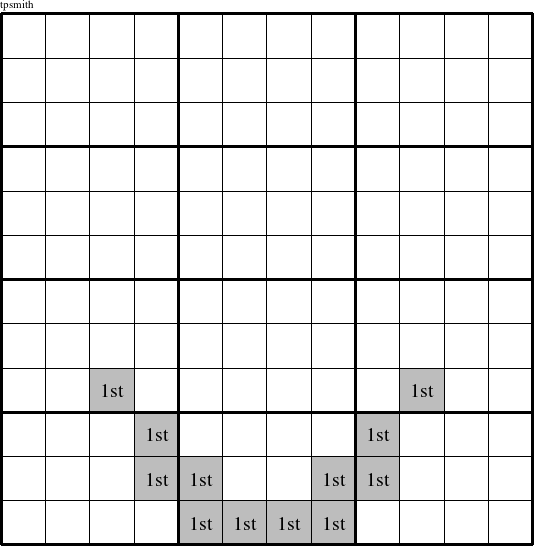In a tpsmith puzzle, the elements that make up the smile are a group and are marked with '1st' in the figure above.